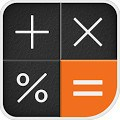# Calculator Memory & Percent APK

Calculator Memory & Percent

Calculator is simple and nicely calculator app designed for Android devices for free.
Designed for everyday calculation, arithmetic operations, calculate percentage.
Simple and intuitive interface for easy to use.
Beautiful display, large button, nicely elegant themes.

Calculation
Basic arithmetic operations.
+ ,-,×,÷

Useful memory is available.

Calculate percentage for tax, statistical, mathematic homework
percentage calculation example:
200 + 8% = 216
200 – 8% = 184
200 × 8% = 16
200 ÷ 8% = 2500

Also Featured
Usefully backspace button to delete the last digit by digit.

Calculation history is saving.
Make single tap the result line for copy to clipboard.
That is so useful.

Calculating taxes, doing homework, and for your everyday.
You can calculate basic calculation, percentage so easily.

#### What’s New

Improved internal process

Name
Calculator Memory & Percent
Package
com.maroyakasoft.dentak
Version
1.1.16b
Size
9.77 MB
Installs